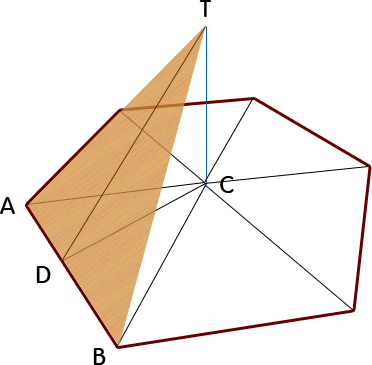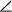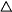SEARCH HOMEMath Central Quandaries & QueriesQuestion from David: Im trying to form a hexagonal pyramid out of plywood and need to know the height to the center point of the pyramid. I want to use standard 4x8 sheets of plywood and split them diagonally. Then with the resulting triangles combine them to form one triangle. Then i want to accumulate 6 of these triangle total and put together to form a hexagonal pyramid.Hi David.

I'll draw one of the triangles on a sketch and label some useful lines and points:According to your plan, AD should be 4 ft and DT should be 8 ft. Therefore AB is also 8 ft.

The length CT is what you want to find.

All the way around a full circle is 360°. Therefore because of symmetry,BCA must be 360°/6 = 60°. Since AC = CB by symmetry, that means all the angles ofBCA are 60, so it is equilateral making AC also equal 8 ft.

If AC is 8 ft and AD is 4 ft, using the Pythagorean Theorem, CD2 = 82 - 42.
But if you look atDCT, it is also a right triangle, so DT2 = CD2 + CT2. This means that
CT2 = DT2 - CD2. But that just means
CT2 = 82 - (82 - 42) = 42.

So CT, the height of the pyramid is 4 ft.

Hope this helps,
Stephen La Rocque.

PS: If you are interested in the angles to cut, then check this earlier question Chris and Harley answered.Math Central is supported by the University of Regina and The Pacific Institute for the Mathematical Sciences.